## Arcanorium CollegeCollege News and Views## 3d Time (7)

Saturday, 15 July 2023 11:14

### TIQM and Hypersphere Cosmology

TIQM and Hypersphere Cosmology.

Abstract.

A conceptual scheme for the unification of the Transactional Interpretation of Quantum Mechanics with Hypersphere Cosmology that may model both repulsive and attractive forces between quanta.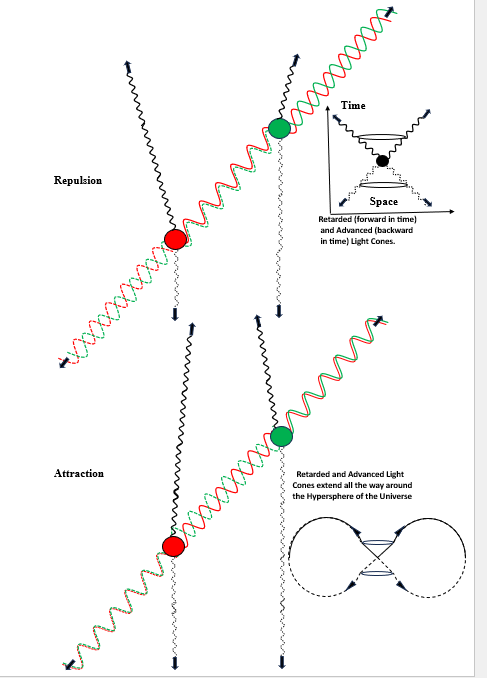Diagrams: -

Upper Right – The Transactional Interpretation of Quantum Mechanics (TIQM) asserts that an energy exchange consists of a ‘quantum handshake’ between an emitter and an absorber in which both emit a retarded (forward in time) and an advanced (backward in time) wave.

We can envisage the so called Null-Paths of the retarded and advanced waves as forming forward and backward  Light Cones where the spacetime interval ((s2 + (ict2))1/2 = 0.

Upper Central – The TIQM model of a photon passing from an emitter (red spot) to a receiver (green spot) with both recoiling.

Note that a ‘complete photon exchange’ has both ‘particle’ and ‘antiparticle’ wave components in phase giving it a spin of twice that of a matter quantum. This event occurs only along a Spacetime Null-Path between the emitter and the absorber. Once established the wave function collapses instantaneously at all points along the null path to complete the exchange of a quantum of energy. Beyond the emitter and the absorber, the wave components lie in antiphase resulting in negation of the wave functions or ‘field excitations’.

TIQM accounts for the observed phenomena of  quantum superposition and entanglement by positing the retro causal effects of advanced waves traveling backwards in time down the emission path to modify the emission conditions.

In TIQM the mutual reinforcement of retarded offer wave and advanced confirmation wave builds up in Pseudo-Time, but it appears instantaneous in ordinary observed time.

It seems reasonable to make two further assertions, firstly that pseudo time lies somehow orthogonal to ordinary observed time, and secondly that so called ‘virtual’ energy ‘particles’ consist of partial quantum handshakes that do not have enough energy to achieve the exchange of a complete quantum of energy.

Such ‘virtual particle’ exchanges dominate much of the structure of the universe in such processes as electromagnetic attraction and repulsion and perhaps also in the action of static gravitational fields.

Examples of repulsive effects – by ‘real’ quanta e.g., photons and perhaps ‘gravitational waves’. By ‘virtual’ photons e.g., electromagnetic repulsion.

Lower Central. – Attractive forces between quanta have always presented a problem of apparent ‘action at a distance’, for how can two objects attract each other if they have to do so by passing something carrying momentum between them? This led to speculation from Newton’s time onwards about Shadow Gravity theories in which a universal mutual ‘push’ between all bodies in the universe becomes shielded by bodies in relative proximity leading to a net attractive effect between them. Such theories however run into problems with thermodynamics.

In the lower central diagram, apparently attractive forces arise between quanta when the retarded and advanced waves between the quanta combine in a state of anti-phase cancellation and the retarded and advanced waves beyond the particles combine reinforcingly in phase to carry momentum away from the quanta in directions that effectively push them towards each other.

Examples of attractive effects – by ‘virtual’ quanta e.g., electromagnetic attraction and perhaps static field gravitational attraction.

Lower Right. – In a Hyperspherical universe the retarded (forward in time) and advanced (backwards in time) lightcones of all matter meet at antipode distance, as do the wave functions of the matter quanta themselves.

We can thus conceptualise attractive effects as arising from repulsive effects acting right round the hypersphere of the universe.

In General. - Quanta exist as ‘Particles’ only fleetingly at the instant of interaction. Between interactions they do not have well defined positions and momenta when traveling as waves in space and time between interaction events.

Ordinary matter owes its apparent constant persistence to highly frequent interactions between its constituent quanta, yet between interactions the constituent quanta do not have counterfactual (unobserved but assumed) definiteness.

Thursday, 01 December 2022 18:18

### The Anti-Singularity Theorems

THE ANTI-SINGLARITY THEOREMS.

We must remain highly suspicious of any theory which asserts that an infinite amount of any physical quantity exists. We can never observe an infinite quantity of anything, and we have no reason to suppose that an infinite quantity of anything can exist. The concept only arises when we introduce it by mistakenly assuming that something else has no limits or by erroneously dividing something by nothing.

If spacetime and mass/energy have a mutual dependency, then we have no reason to assume that spacetime exists independently and infinitely beyond the presence of mass/energy.

Contemporary theoretical physics suffers from the delusion that mass/energy can become concentrated in an arbitrarily small volume, including zero volume. This has led to the assumption that black holes must contain singularities and that this universe arose from a zero dimensional singularity and the assumption that fundamental quanta can exist as zero dimensional point particles.

The Schwarzschild  Solution of General Relativity: -

r = 2Gm/c^2 shows that an event horizon at radius r will arise when a given mass m collapses to that radius under its own gravity creating a black hole.

The Penrose-Hawking Singularity Theorems assert that nothing can prevent further collapse and thus that a spacetime singularity consisting of a zero-dimensional point of infinite density must inevitably form within any black hole.

The Godel Solution of General Relativity: -

w = 2sqrt(piGp) does however indicate that something will prevent the collapse of matter into spacetime singularities.

‘Matter everywhere rotates relative to the compass of inertia with an angular velocity (w) of twice the square root of pi times the gravitational constant (G) times the density (p)’.

As matter becomes denser, spacetime curvature increases and a compact body acquires a radius excess which gives it more volume on the inside than an outside observer would expect from its exterior radius by assuming 4/3 pi r^3 for flat Euclidian space.

When a body contracts to r = 2Gm/pi c^2  (or pi r = 2Gm/c^2) the radius excess makes its internal ‘diameter’ equal to half of its circumference and the body becomes a Glome Hypersphere. With a volume of 2pi^2 r^3 or 2L^3/pi where L means antipode distance.

The Godel Metric w = 2sqrt(piGp) then supplies the internal metric of such hyperspheres in the reference frame of internal observers, and it shows that such bodies must have an angular velocity w.

Substituting hypersphere volume into the Godel metric shows that a Glome hypersphere will rotate with an angular velocity of pi c/L and hence a frequency f of c/2L and have balanced centripetal and centrifugal accelerations a of c^2/L.

Given that: -    w = 2sqrt(piGp)w = 2pi f,   p = mass m/volume v,    2Gm/L = c^2

And that hypersphere volume v = 2L^3/pi

Then: -    w = 2sqrt(piGp),         w^2 = 4pi^2Gm/2L^3,            w^2 = pi^2c^2/L^2,

w = pi c/L,        2pi f = pi c/L,      f = c/2L,    a = c^2/L.

The mass inside a Glome hypersphere does not rotate as a simple sphere with a single axis of rotation, but rather it rotates about the randomly orientated axes of the Hopf Fibration.

Hence such Glome Hyperspheres cannot contract any further because that would cause them to rotate faster than light. This falsifies the Penrose-Hawking Singularity Theorems for both black holes and the universe as a whole.

If the mass of the universe remains constant, then so does its size, despite appearances. (See the Hypersphere Cosmology redshift-distance and hyperspherical lensing relationships.)

Now if such hypersphere mechanics forbids the formation of spacetime singularities at cosmic and astronomical scales then it most likely also forbids the formation of fundamental zero dimensional point particles. Yet we cannot usefully regard quanta as miniature cannon balls.

Quanta also have characteristic wavelengths many orders of magnitude greater than the antipode lengths suggested by L = 2Gm/c^2 for such particles. Yet the wavelengths of quanta have a directional quality which we most commonly observe in the direction of propagation rather than transverse to it.

The following calculation shows that given the Planck-Einstein relationship E = hf and the Godel metric w = 2sqrt(piGp), quanta with mass have a definite volume: -

Given that: -  E = hf,    w = 2sqrt(piGd),   w = 2pi f,   E = mc^2,   p = mass m/volume v

Then: -      f = mc^2/h,         w = 2pi mc^2/h,         2pi mc^2/h = 2sqrt(piGm/v),

pi^2 m^2c^4/h^2 = piGm/v,       pi mc^4/h^2 = G/v,

v = Gh^2/pi mc^4

This closely matches the Einstein-Cartan volume of a quantum ~ Gh^2/mc^4  (derived by other means).

Thus, it seems probable that quanta embody a hyper-geometrical component in their probably variable dynamic geometry, rather than existing as simple 3-sphere Glome Hyperspheres. Rotating Hypertoruses of some kind may supply a solution. Research continues.

As quanta have a spatial volume, then that volume sets yet another limit to the compressibility of matter and its maximum possible density. This also sets a minimum bound for the mass of any black hole.

Tuesday, 01 March 2022 20:03

### Algebrology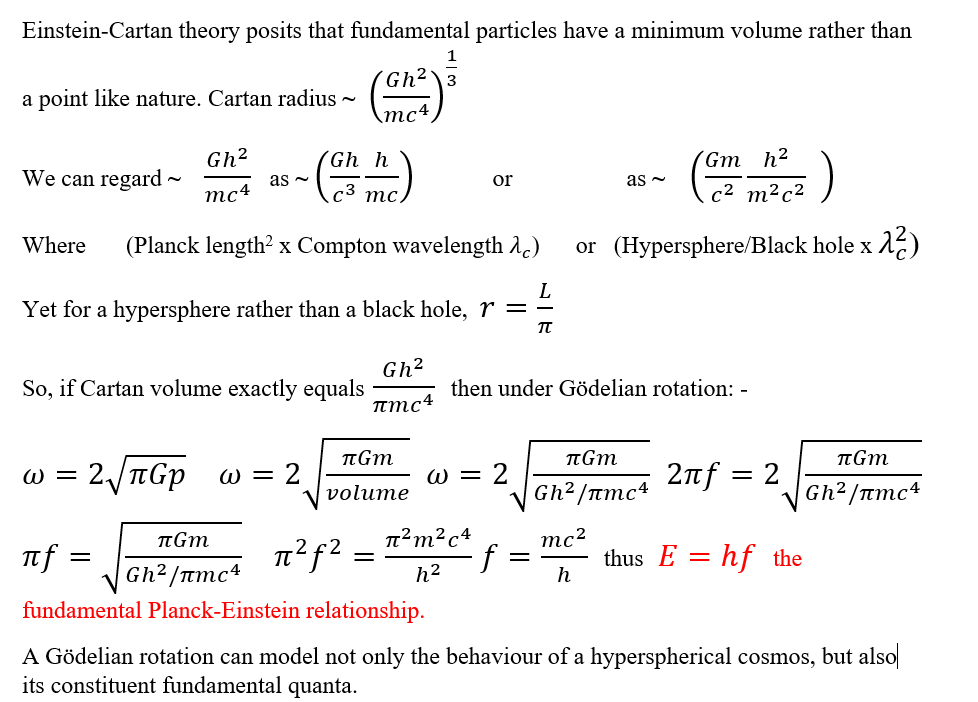Wednesday, 12 January 2022 13:42

### Quantum Principle of Relativity

Quantum Principle of Relativity

This recent paper by Andrzej Dragan and Artur Ekert : -

appears to derive some of the basic strangeness of quantum mechanics from a consideration of the conventionally discarded superluminal (faster than light) solutions of Special Relativity. In particular, the Lorentz transformations suggest that in a superluminal reference frame, the dimensional signatures undergo sign reversal changing space into time and time into space.

The Dragan & Ekert paper also suggests that incorporating both the subluminal and superluminal reference frames explains the apparent indeterminacy, the multiple paths, and the Huygens wavefront phenomena of quantum mechanics, whilst positing additional time-like dimensions.

Now the Transactional Interpretation of Quantum Mechanics similarly does not discard the Advanced Wave solutions to Maxwell’s equations of electromagnetism, and it explains quantum mechanics in terms of advanced waves traveling ‘superluminally’ backwards in time and retarded waves traveling forwards in time.

Some interpretations of quantum mechanics describe antiparticles as time-reversed particles, and bosons as particle-antiparticle pairs.

A full inclusion of frames of reference on both sides of the lightspeed barrier seems to lead to a Six-Dimensional Quantum Spacetime.

Spacetime Metric, where  + = space,  - = time.

Dimensions (Minkowski)

Subluminal Retarded Wave (R)             +R  +R  +R  -R   (3 space, 1 time)

Superluminal Advanced Wave (A)        +A   -A   -A  -A  (1 space, 3 time)

Overall result (Subluminal reference frame)

Space,  1, +R +A,   Direction of travel, forwards or backwards. X axis.

Space,  2,   +R. Orthogonal to direction of travel. Y axis.

Space,  3,   +R, Orthogonal to direction of travel. Z axis.

Time,    1,  -R -A, Direction of travel, forwards or backwards.

Time,    2,   -A, Orthogonal to ordinary time, imaginary time or ‘pseudo-space’.

Time,    3,   -A,  Orthogonal to ordinary time, imaginary time or ‘pseudo-space’.

Further, it is interesting to note that within the subluminal reference frame only quanta carrying imaginary time pseudo spatial spins 2 and 3, responsible for electric and nuclear charges, have mass and sub-lightspeed velocities, whilst photons and neutrinos which lack these spins always travel at lightspeed.

Work continues........

Monday, 08 November 2021 19:37

### Delayed Choice Quantum Eraser Ansible

Delayed Choice Quantum Eraser Ansible.

The following thought experiment shows that in principle instantaneous communication remains possible over any distance, including interstellar and intergalactic distance; and further that this need not lead to paradoxes or causality violations in a universe with finite a lightspeed; and further that something akin to a cosmic internet probably already exists.

Consider what a delayed choice quantum eraser can do: -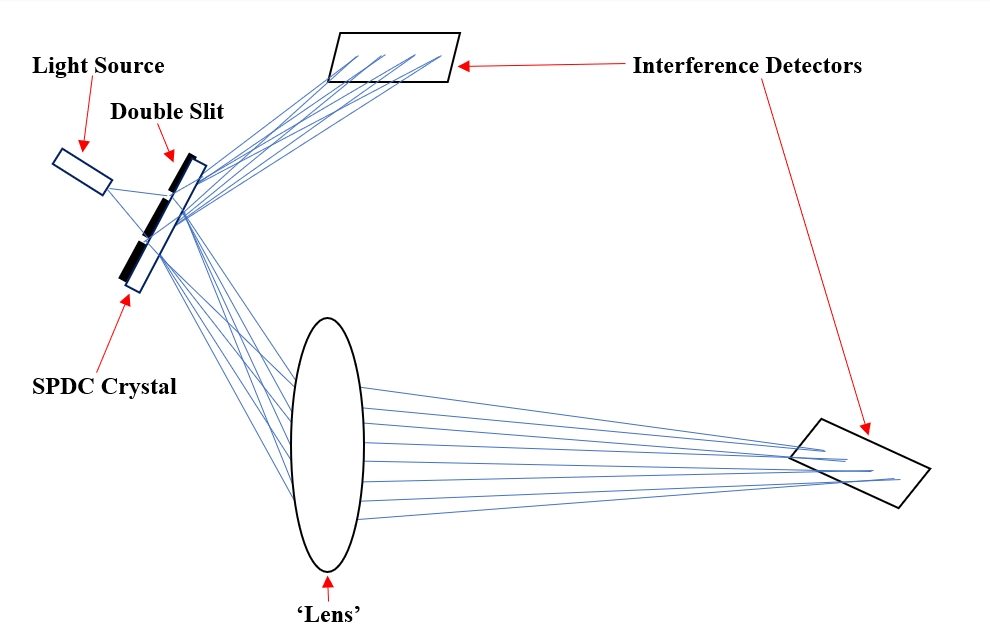Light from the Source passes through a Double Slit. The Spontaneous Parametric Down Converter Crystal splits each quantum of light into two Entangled quanta, each of half the energy of the original, and these quanta follow divergent paths.

The quanta which diverge upwards meet at a nearby Interference Detector which could in principle consist of a simple screen. The quanta which diverge downwards go through a suitably sophisticated ‘lens’ arrangement which can focus them at any distance including theoretical infinity.

Because of the entanglement between the quanta  which go to each of the separate detectors, any action at either detector site which destroys the interference pattern will instantaneously destroy the interference pattern at the other site no matter how far apart they lie.

Simply turning one of the Interference Detectors on and then off will cause the other detector to show an on and then an off signal and thus the whole arrangement can transmit binary code instantaneously over any distance.

This need not lead to paradoxes or causality violations in a universe with finite a lightspeed.

Imagine two groups of aliens, A and B, living on planets a hundred light years distant from each other. When A receives light from B it knows that the light left B a hundred years ago, and when B receives light from A it knows that light left A a hundred years ago. If either A or B tries to send an ordinary lightspeed message to the other, it will take a hundred years to get there, and a spaceship journey will take at least a hundred years at minimum.

However, if either A or B detects Ansible light from the other and decides to modulate its reception by alternately directing it into an interference detector and not doing so, then the other will notice a signal at the time at which they sent the Ansible light.

Thus, it can appear as if each party sends a signal back into the past, but the ‘past’ to which they send it lies a hundred light years away in space, so the space and time cancel each other out.

d = sqrt(s2 + (ict)2(Minkowski, after Pythagoras.)

Absolute spacetime distance = sqrt(space2 – (lightspeed x time)2 )This can equal zero.

In practise if two alien civilisations, separated by a hundred light years, simultaneously came up with the idea of Ansibles and both guessed the same most likely frequencies to use, then they could both start exchanging binary code immediately. They would not have to wait for a hundred years for the Ansible light to pass between them.

According to  the current standard cosmology, life has had over ten billion years to develop in the universe. According to Hypersphere Cosmology it has had unlimited time. In the first case a universe wide Ansible network probably already exists, in the second case it almost certainly does.

We just need to hack into it and decode it. That, rather than the discovery of Faster Than Light drive, may constitute the real entry requirement to interstellar society.

Wednesday, 02 June 2021 21:27

### Particle Spins

Unlike the Hypersphere Cosmology model on this site which has achieved maturity and a fully quantitative exigesis, this investigation into the quantum microcosm remains speculative, experimental, qualitative, and a work in progress. All criticism and collaboration welcomed by email.

Descriptions and Explanations. Any Explanation of phenomena depends on Descriptions of underlying phenomena. Thus, for example, we explain chemistry in terms of atoms, and we explain atoms in terms of quantum physics. However, we have no satisfactory description of the phenomena underlying quantum physics yet. These musings experiment with descriptions of possible spacetime geometries underlying quanta and quantum fields. Any such description should explain the existence and behaviour of known particles and fields and if possible, make novel predictions.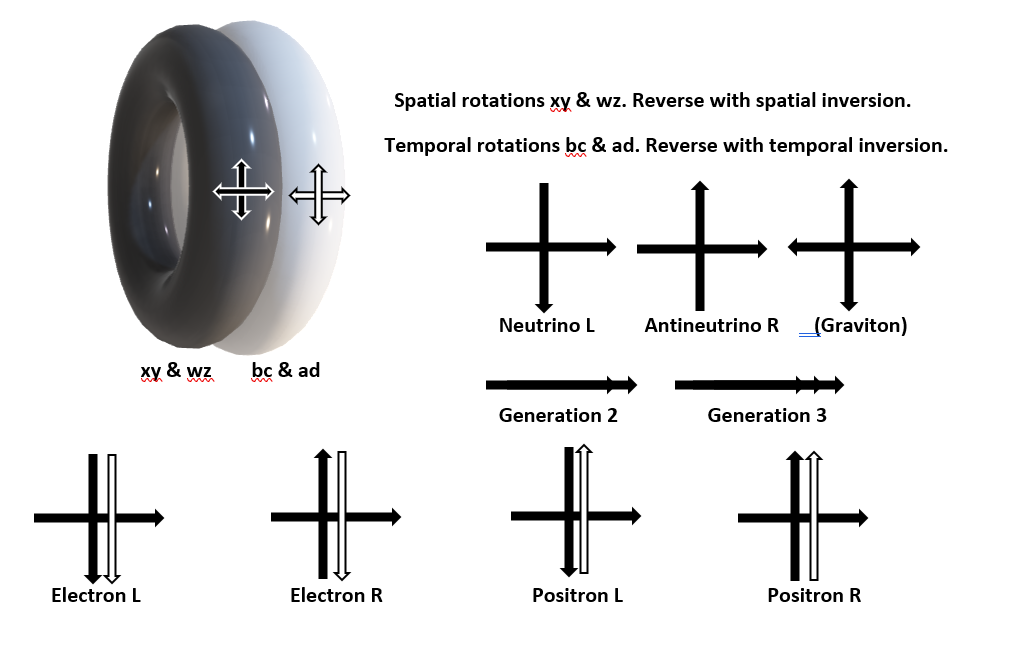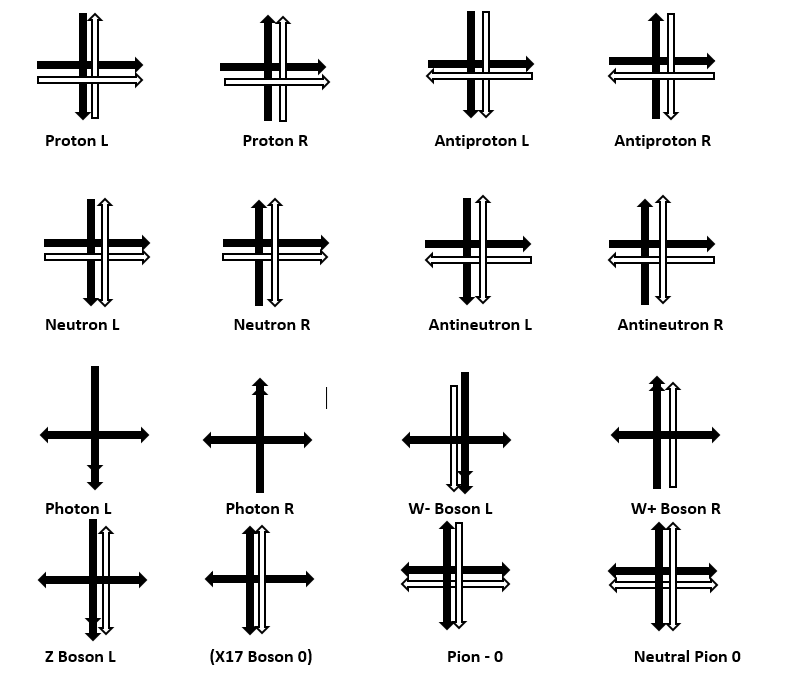Table of fundamenal particles modelled as toruses.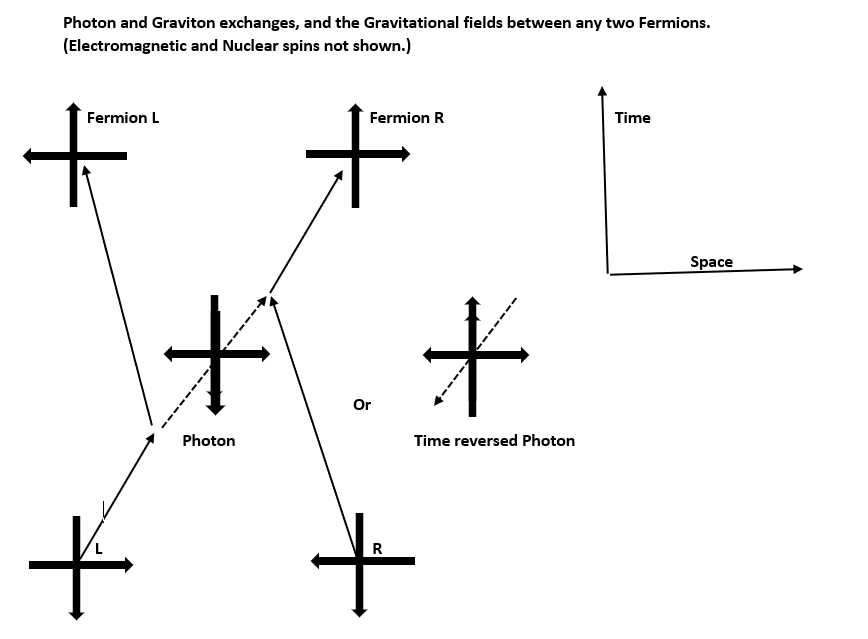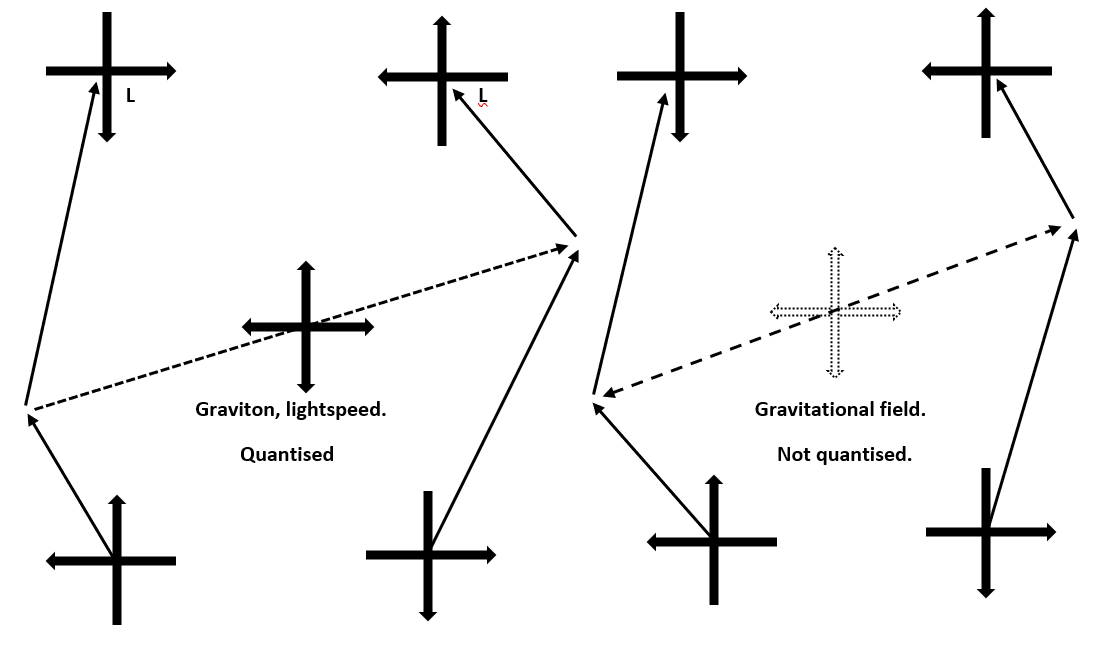Table of simple partice interactions

This model begins with the simplest quantum, that of the neutrino, modelled as a spinor based on a Clifford Torus that rotates twice in the toroidal mode for each poloidal rotation.

This spinorial rotation allows for only two orientations of the poloidal rotation with respect to the direction of travel which lies orthogonal to it as neutrinos always travel at lightspeed. These two orientations correspond to neutrino (left) and antineutrino (right).

This spinorial rotation subtends a wavelike series of twists throughout all spacetime that extends across the universe and creates a field surrounding the particle.

Charged quanta result from rotations involving the pseudo-spatial plane of imaginary time, poloidal rotations give rise to positive and negative electric charges and toroidal rotations give rise to positive and negative nuclear charges. These also subtend wavelike series of twists throughout all spacetime that extends across the universe and create fields surrounding the quantum.

Quanta do not have a zero-dimensional point-like geometry, they consist of Clifford Tori with a similar topology to Hyperspheres (3-Spheres) except that they have a directionality of spins and propagation.

Quanta arise where incoming waves returning from the rest of the universe undergo a spinorial 3-Rotation and become outgoing waves. Spacetime consists of a single field which can carry several different types of twist or wave.

Electrostatic and Gravitational fields effectively act across any distance but with an effect that diminishes with the inverse square of distance. Fields rather than quantised particles mediate these interactions. Virtual non-quantised ‘particles’ do not exist, although images of them may prove useful in visualising the field geometry.

Photons consist of quanta of spins that become exchanged between fermions, these always propagate at lightspeed and always have a repulsive effect with both fermions recoiling. They do not carry electric charge and neither do electric fields affect them. We only regard them as the quanta of electromagnetism because we only observe their interactions between charge carrying fermions.

Gravitons appear as gravity waves when enormous masses accelerate, and they propagate at lightspeed with an exclusively attractive effect. Ordinary gravity works via fields.

Exchange particles such as photons and gravitons and all other bosons have a particle-antiparticle configuration, the exchange of such quanta occurs as a quantum handshake as in the Transactional Interpretation of Quantum Mechanics. Field effects arise in the same way although at the lower energies involved they do not give rise to quantised boson particles.

This model accommodates an additional charged boson to complement the W+, W-, and Z. This X17 boson has probably already appeared in the decay of Beryllium 8.

In this model all spins remain conserved on the basis that + and – equals 0, allowing for both particle creation and annihilation, energy permitting. Generation or ‘flavour’ remains conserved over a complete cycle of particle-antiparticle creation and subsequent decays.

The whole of QCD becomes discarded, gluons do not exist. Exotic transiently stable baryons and mesons simply have extra spins about the basic baryon or baryon-antibaryon configurations.

The mass-energy equivalence and the equivalence of inertial and gravitational mass arise from the effect of all the other spins upon the xy/wz spinors of quanta.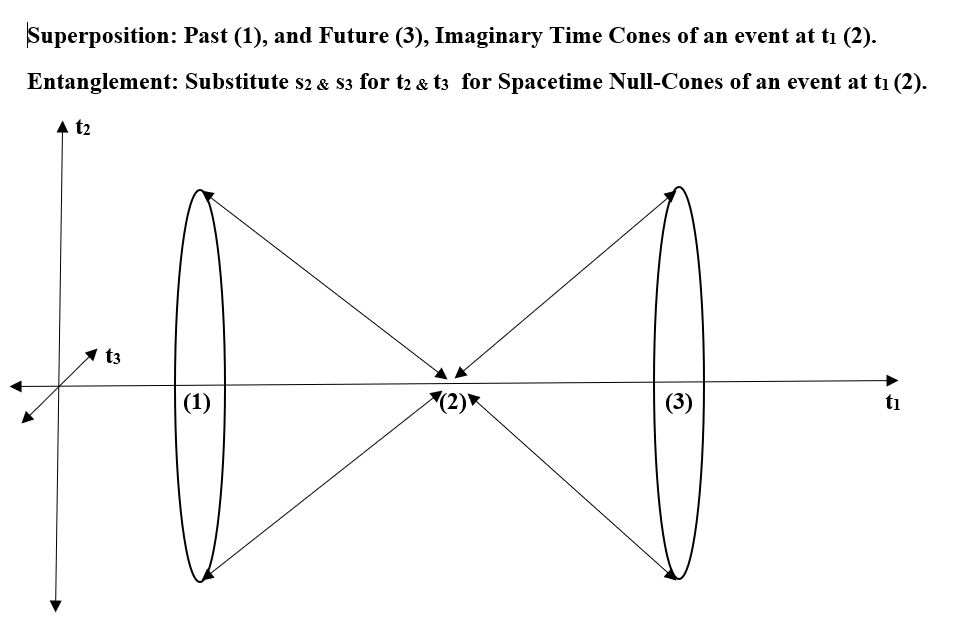All particles extend fields right around the hypersphere of the universe, these converge at antiparticles at their antipodes. This explains the apparent lack of antimatter in the universe.

In/Out waves of spin converge and undergo 3-rotation to make non-point particles of finite size.

Every particle depends for its properties on the whole of the rest of the universe.

The Ubiquity Constant and the quantisation scale depend somehow on the macrostructure of the universe.

Quantised ‘kinks’ in space can only move at lightspeed, or less if they embody the temporal electric or nuclear spins which confer mass.

The Higgs mechanism does not apply. If the tiny 125 GeV blip corresponds to anything it probably corresponds to a transient  ZZ di-boson.

Volume = 2pi2r3 for both a hypersphere and a (Clifford?) horn torus.

Does the local field geometry change from a vorticitating 3-Hypersphere to two rotating 3-Tori upon particle-antiparticle pair production and thus confer particle directionality?

Can Macrostructures modulate waves to carry structural information?

If so, all manner of psi effects and morphic field effects become possible and the universe will exhibit Stochastic Holism.

Three Dimensional Time Resources.

Herewith a selection of some of the main papers on 3D Time and SO(3,3) spacetime.

Herewith a fascinating piece of speculation from Gerard 't Hooft

See the 'fast variables' and multidimensional toroids.

Thursday, 25 October 2018 20:56

### Mach's Principle

Abstract.

This paper presents a quantitative equation for Mach’s Principle that appears to satisfy the requirements of most of the qualitative expressions of Mach’s Principle, and it suggests that the universe has spatial closure and a Transactional gravitational exchange mechanism.

Mach’s Principle.

We recognise mass as having two familiar components, firstly inertial mass – a resistance to acceleration as quantified by Newton’s second law that force equals mass times acceleration, shown here by denoting the mass involved as the inertial mass.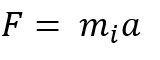Secondly mass also exhibits a gravitational effect, any two masses appear to attract each other with a force described by Newton’s equation of gravity. In this, the force between a mass m (usually a large mass like a planet or a star) and the mass of interest depends on both masses, the gravitational constant G, and the square of the distance between them. He we denote the mass of interest (which usually means the smaller one that falls or moves most) by its gravitational massNow in Newton’s theory, inertial mass  miraculously seems to exactly equal gravitational mass  and this equivalence explains Galileo’s observation that all masses fall at the same rate.  Thus although a heavier mass has more inertia and needs more force to move it, its greater mass gives gravity an exactly proportional extra amount of gravitational mass to work on, so to speak, so all masses fall at the same speed.

On the human scale, inertial mass often seems intuitively ‘stronger’ than gravitational mass, we can feel the resistance a cannon ball offers to our pushing it around, but we cannot feel the gravitational attraction between it and ourselves. This arises because the ‘ratio’ between gravitational mass and inertial mass has a very small value represented by G, the gravitational constant. Nevertheless, the ratio remains constant and an amount of material that has a certain amount of inertia will always have a certain corresponding amount of gravity.

Now Einstein saw something deeper and beyond mere convenient coincidence in the equivalence of inertial and gravitational mass (or more precisely in the equivalence of acceleration and gravity), and he reformulated our ideas about gravity.

He described gravity not as some sort of force at a distance but as an effect arising from the curvature of spacetime by mass. Thus, a massive object like a planet curves spacetime about itself and freely moving objects all follow this curvature by falling towards it at the same rate, and they do not actually feel any forces at all whilst in free fall.

‘Spacetime tells matter how to move; matter tells spacetime how to curve.’ As John Archibald Wheeler so elegantly summarised the idea of General Relativity.

The description of gravity as spacetime curvature gives far more accurate predictions than the basic Newtonian model but it does not say much about the inertial component of mass. In the Newtonian model, inertial mass just appears as somehow intrinsic to an object and gravity appears as a mysterious ‘force at a distance’. Neither of these ideas sits comfortably with the relativistic perspective in which properties arise on a relational basis.

Mach's Principle has hung around on the fringes of cosmology and relativity for 100 years, it has influenced a number of theorists but nobody seems to have managed to formulate it concisely or to develop some maths for it. Roughly speaking it suggests that the inertial mass of any object arises because of the effect of all the stuff in the entire universe, so as Mach put it, if the subway jerks, the far stars and galaxies throw you to the ground

This idea has lurked within physics since Einstein named it after some remarks by Mach (who devised the Mach numbers for multiples of the speed of sound). Nobody appears to have found a way to quantify it and various qualitative versions of it exist. It broadly states that some relationship should exist between the inertia of any body and ALL of the rest of the material in the entire universe. This would seem to imply a spatially closed universe, otherwise inertial mass, and many of the laws of physics, would vary with time.

Debate rages about whether General Relativity really incorporates Mach’s Principle or not. The currently most popular interpretation of cosmological observations asserts that the universe expands from some sort of a big bang, in which case Mach’s Principle seems invalid unless inertial masses either vary with time or remain invariant to the distribution of matter within the universe. As it appears that the distribution of matter in the universe always remains homogenous on a large scale, this distribution invariance comes down to an invariance to the size of the universe which seems highly unlikely as  the spacetime curvature we recognise as gravity remains very much distance dependent.

Now if the inertia of any object depends on the entire rest of the universe then it must depend on the gravitational mass of the body in question, the gravitational mass of the entire universe, the Gravitational constant that relates them, and the size of the universe, and the speed of light. Moreover, the ratio of inertial to gravitational mass must remain constant as we have every indication from astronomy that it has not varied over the observable history of the universe.

Now in Hypersphere Cosmology1 , which posits a non-expanding universe: -Where G = The Gravitational constant

M = The Mass of the universe

L = The antipode distance in a hyperspherical universe

c = The speed of light.

This strongly suggests that the following equation quantitatively fulfils the major qualitative requirements of most expressions of Mach’s Principle.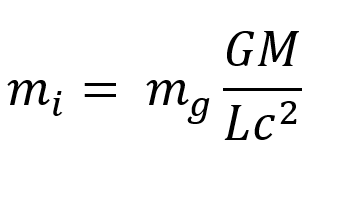If the gravitational constant of the universe or the mass of the universe were to increase so would all inertial masses, conversely any increment in the size of the universe or lightspeed would decrease all inertial masses.

GM/Lc^2 thus appears as a sort of scalar field, omnipresent and apparently non-local* in the hypersphere of the universe, and giving rise to an acceleration A = GM/L^2 which has detectable effects such as the Pioneer anomaly and the anomalous galactic rotation curves.

(See Hypersphere Cosmology1 )

At present the equation has no practical use except to complete hypersphere cosmology because there seems no way we could currently manipulate the effects of G,M,L, or c, in the vicinity of a spacecraft, but if we could reduce inertial mass somehow then we could easily move matter around the universe.

*The apparently instantaneous effects of both inertia and ‘static’ gravitational spacetime curvatures raises interesting questions. Gravitational waves undoubtedly propagate at light-speed from accelerated masses as Einstein predicted and as recent experiment has confirmed.

If we accept that nothing can travel faster than light then the apparently instantaneous effects of inertia and static spacetime curvatures, (gravity ‘fields’) may arise from a Transactional Exchange between curvatures. The laws of gravity seem completely time symmetric and capable of supporting advanced negative curvatures propagating down retarded positive curvature paths back into the past to create apparently instantaneous effects.

The time-symmetric nature of all physical laws except the Second Law of Thermodynamics (which states that in an isolated system Entropy will increase only) at first appears very mysterious and physicists usually quietly ignore the time reversed solutions to their equations and calculations.

Hawkin remarked that ‘Entropy increases with Time because we measure Time in the direction in which Entropy increases’. We have no way of telling in which ‘direction’ ‘time goes’ or even if it actually ‘goes’ anywhere.

If however, we adopt the Transactional Interpretation and extend it beyond quantum physics to gravitation and to model gravitational effects as arising from positive spacetime curvatures propagating forward in time and negative spatial curvatures propagating backwards from the future to create effects in the present then we can explain the apparently instantaneous effects of inertia and static gravitational ‘fields’.

Of course, the same argument applies to electrostatic fields that would then correspond to a special class of spacetime curvatures.

Accelerations of masses and electrostatic charges will of course create disturbances in spacetime curvatures that propagate at light speed as bosons with the wave –particle duality characteristic of quanta. However, the concept of virtual bosons mediating static gravitational and electrostatic interactions becomes redundant.

Reference1.

Hypersphere Cosmology 2. Author: Peter J Carroll. http://vixra.org/abs/1601.0026

• #### Arcanorium College - Department of Science. +

The Arcanorium College Department of Science, Research and Collaboration Facility.   ‘Nobody understands Quantum Physics’, as Richard Feynman observed, and
• #### Arcanorium College - Department of Magic. +

The Arcanorium College Department of Magic, Degree in Magic. Few if any academic institutions in the known universe recognise the
• #### Arcanorium College +Arcanorium College Interroga Omnia – Question all things. Arcanorium College consists of a Natural Philosophy Faculty with two Departments: - The Department
• 1